Next: Simple Harmonic Motion Up: One-Dimensional Motion Previous: Motion in a General

# Velocity Dependent Forces

Consider a particle of mass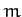moving in one dimension under the action of a force,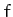, which is a function of the particle's speed,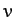, but not of its displacement,. Note that such a force is intrinsically non-conservative [since it clearly cannot be expressed as minus the gradient of some potential function,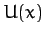]. Now, the particle's equation of motion is written(56)

Integrating this equation, we obtain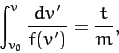(57)

where. In principle, the above equation can be solved to give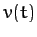. The equation of motion is also written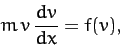(58)

since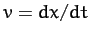. Integrating this equation, we obtain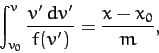(59)

where. In principle, the above equation can be solved to give.

Let us now consider a specific example. Suppose that an object of massfalls vertically under gravity. Letbe the height through which the object has fallen since, at which time the object is assumed to be at rest. It follows that. Suppose that, in addition to the force of gravity,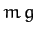, where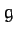is the gravitational acceleration, our object is subject to a retarding air resistance force which is proportional to the square of its instantaneous velocity. The object's equation of motion is thus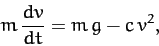(60)

where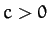. This equation can be integrated to give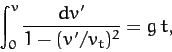(61)

where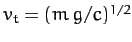. Making a change of variable, we obtain(62)

The left-hand side of the above equation is now a standard integral, which can be solved to give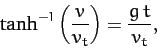(63)

or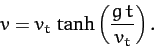(64)

Thus, when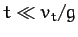, we obtain the standard result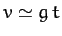, since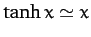for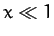. However, when, we get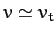, since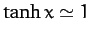for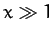. It follows that air resistance prevents the downward velocity of our object from increasing indefinitely as it falls. Instead, at large times, the velocity asymptotically approaches the so-called terminal velocity,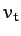(at which the gravitational and air resistance forces balance).

The equation of motion of our falling object is also written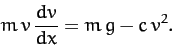(65)

This equation can be integrated to give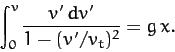(66)

Making a change of variable, we obtain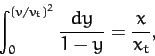(67)

where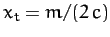. The left-hand side of the above equation is now a standard integral, which can be solved to give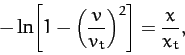(68)

or(69)

It follows that our object needs to fall a distance of order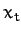before it achieves its terminal velocity.

Incidentally, it is quite easy to account for an air resistance force which scales as the square of projectile velocity. Let us imaging that our projectile is moving sufficiently rapidly that air does not have enough time to flow around it, and is instead simply knocked out of the way. If our projectile has cross-sectional area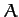, perpendicular to the direction of its motion, and is moving with speed, then the mass of air that it knocks out of its way per second is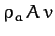, whereis the mass density of air. Suppose that the air knocked out of the way is pushed in the direction of the projectile's motion with a speed of order. It follows that the air gains momentum per unit timein the direction of the projectile's motion. Hence, by Newton's third law, the projectile loses the same momentum per unit time in the direction of its motion. In other words, the projectile is subject to a drag force of magnitude(70)

acting in the opposite direction to its motion. Here,is an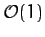dimensionless constant, known as the drag coefficient, which depends on the exact shape of the projectile. Obviously, streamlined projectiles, such as arrows, have small drag coefficients, whereas non-streamlined projectiles, such as bricks, have large drag coefficients. From before, the terminal velocity of our projectile is, whereis its mass, and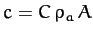. Writing, where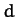is the typical linear dimension of the projectile, andits mass density, we obtain(71)

The above expression tells us that large, dense, streamlined projectiles (e.g., medicine balls) tend to have large terminal velocities, and small, rarefied, non-streamlined projectiles (e.g., feathers) tend to have small terminal velocities. Hence, the former type of projectile is relatively less affected by air resistance than the latter.Next: Simple Harmonic Motion Up: One-Dimensional Motion Previous: Motion in a General
Richard Fitzpatrick 2011-03-31